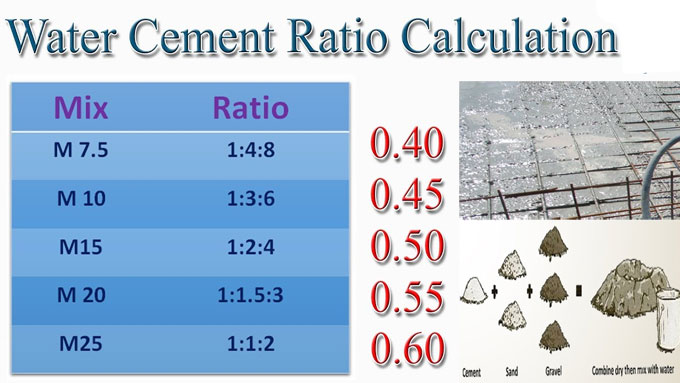# Calculation of water cement ratio in concreteDefinition Of Water Cement Ratio - Water Cement Ratio indicates the ratio among the weight of water to the weight of cement applied in concrete mix.

Generally water cement ratio remains under 0.4 to 0.6 according to IS Code 10262 (2009) for nominal mix (M10, M15 ?. M25).

The strength of concrete is directly influenced by water cement ratio. When, the water cement ratio is perfect, the strength of concrete is raised otherwise the strength will be reduced.

Role of Water in Concrete: Concrete belongs to a macro content. It comprises of micro elements like cement, sand, fine aggregate & Coarse aggregate. To produce high strength concrete to resist necessary compressive strength, the ratio of admixture should be perfect to mix these materials.

The water can accelerate this chemical process with the addition of 23%-25% of the cement volume. Besides, it creates 15% of water cement paste defined as gel to fill up the voids in the concrete.

Impacts of excessive water in concrete: 23% of water is required to begin the chemical process on cement.

Inclusion of extra water than this permissible water cement limit will actually influence the strength.

If the process is going on to add water for enhancing the workability then the concrete contains various fluid materials where the aggregates will set. As soon as the water is vaporized it produces several voids in concrete and as a result the concrete strength is reduced.

Workability Of Concrete: Workability signifies the capacity of concrete to manage, transmit and place devoid of any segregation. The concrete becomes effective if it can be simply operated, placed and transmitted devoid of any segregation to be arranged in the job site.

Computation of Water Cement Ratio: Actually, water cement ratio is chosen from different workability test depending on the structural members, concrete strength, transportation, selection of aggregation etc.

Computation of Water Quantity for Concrete: The W/C Ratio differs from 0.4 to 0.7 on the basis of exposure conditions.

If it is required to measure the water quantity for concrete, initially determine the cement content for the volume.

Suppose, the desired cement volume is 50kg.
Required amount of water = W/C Ratio X Cement Volume

So, required amount of water = 0.5 X 50 kg = 25 litres / 50 kg cement bag.

For Design mix, the W/C ratio is based on the workability, strength requirements.